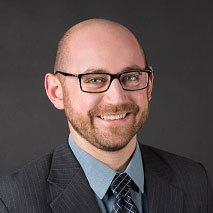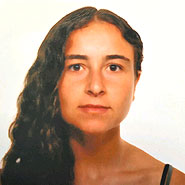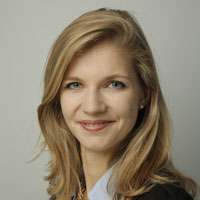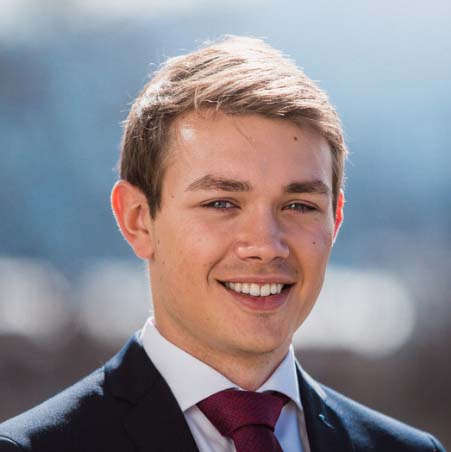# Quantum technology & computing

Quantum computers have the potential to solve certain problems more efficiently than classical computers. In recent years, research on quantum computing hardware has made significant progress, and first devices are now accessible to the public through the cloud. Therefore, studying applications of quantum computers is becoming increasingly important to leverage their full potential once more powerful machines are available. In that respect, it is crucial to understand the timeline to quantum advantage and business impact. Our group is developing and analyzing new quantum algorithms for a wide range of applications, such as quantum chemistry as well as optimization and machine-learning algorithms for finance or supply-chain management.

## Quantum chemistry

Recent advances in the field of quantum computing have ignited the hope that one day we might be able to solve complex materials-science problems using quantum computers. The direct mapping of the molecular wave function to the qubit state allows the implementation of all quantum operations into a number of gates that only scales polynomially with system size. A quantum computer could then be used to simulate chemical systems and their properties, including correlation functions and reaction rates. What’s more, a small quantum computer comprising on the order of 100 qubits will be able to address challenging problems in quantum chemistry that are beyond the reach of classical algorithms. Improving quantum algorithms, such as by reducing the number of gate operations required, might further facilitate this task.

## New quantum (approximation) algorithms

#### Solving electronic structure problems 20–100 qubits

Observables
Ground and excited-state energies

[ More ]

Solving electronic structure problems (20–100 qubits)

Development of quantum algorithms to calculate the electronic structure properties of molecular and solid-state systems. These include the ground and excited-state energies as well as a number of other quantities of potential interest in physics, chemistry and biology.

#### Derive molecular dynamics schemes, including forces

100–500 qubits, longer circuits

[ More ]

Derive molecular dynamics schemes, including forces

The approaches derived to calculate the electronic structure properties of molecular systems can be extended to the calculation of molecular forces to optimize molecular geometries. Ultimately, the forces will also be used in molecular dynamics to simulate chemical processes or to sample the configuration space.

#### Exploring chemical and configuration space of molecules and materials

50–200 qubits

[ More ]

Exploring chemical and configuration space of molecules and materials

The search for new molecules with tailored properties in the exponentially large chemical space is hampered by the unfavorable scaling of classical algorithms. We are currently working on a new class of quantum algorithms that can perform an efficient search in the chemical compound space. Similar approaches will also be applied to optimize chemical structures in configuration space, for example to solve the protein folding problem.

#### Optimizing hardware (gates) for quantum chemistry applications

Pulse shaping using local control theory

[ More ]

Optimizing hardware (gates) for quantum chemistry applications

The different gate operations used in quantum circuits can be optimized by means of numerical simulations of the different components (qubits, couplers and gate pulses). Our aim is to derive new theoretical frameworks for the simulation of one- and two-qubit operations for future implementation in real hardware. Currently, our focus is on improving the tunable coupler gate fidelity using approaches to characterize the noise sources and pulse-haping techniques.

## Quantum optimization and machine learning

Applications of optimization and machine-learning algorithms are appearing in every industry, sometimes with enormous potential. For example, they can reduce costs, accelerate processes, or reduce risk. In practice, input data for most optimization problems is not fully deterministic, but is at least partially uncertain, which makes these problems even more complex to solve.

There are good reasons to believe that quantum computers can help solve complicated optimization problems, with and without uncertainty. Quantum heuristics exist for combinatorial optimization; there are quantum algorithms to solve systems of linear equations or semi-definite programs, and quantum computers can naturally represent random distributions in the form of superposition states.

Our group is developing, analyzing, and applying new quantum algorithms to relevant optimization problems, for example in finance and supply-chain management. In addition, we are endeavoring to find new applications where quantum computers could lead to an advantage in the future.

## Applications of quantum optimization and machine learning

#### Combinatorial optimization

Portfolio optimization, scheduling, protein folding

[ More ]

Combinatorial optimization

Combinatorial optimization problems have applications ranging from routing and scheduling to circuit design and protein or polymer folding. However, the problem complexity usually scales exponentially with the problem size. Quantum computers have the potential to approximate good solutions to such problems faster than classical computers. We are developing efficient models and analyzing the performance of quantum algorithms to solve such problems.

#### Solving partial differential equations

Finite element / Difference methods
Variational methods

[ More ]

Solving partial differential equations

Partial differential equations (PDEs) can model a large variety of phenomena, such as heat, fluid dynamics and quantum mechanics. Algorithms to approximate solutions of PDEs are often based on solving systems of linear equations, where quantum computers potentially lead to a significant performance improvement. We are studying efficient quantum representations of specific problems as well as developing and analyzing new algorithms.

#### Quantum machine learning

Supervised learning and classification

[ More ]

Quantum machine learning

Quantum computers can represent quantum networks with an exponentially large number of qubits. Such networks can be used to develop new kinds of classifiers, or approximation architectures, for supervised learning. We are analyzing such networks and comparing their performance with respect to classical classifiers.

#### Optimization under uncertainty

Risk analysis (finance)
Dynamic processes (supply chains)

[ More ]

Optimization under uncertainty

Many business optimization problems involve uncertainty, for example with regard to prices, interest rates, or customer demand. Problems of practical interest include determining optimal order policies or production schedules in supply-chain management, risk evaluation of financial products, or portfolio optimization, which is also a combinatorial optimization problem. Quantum computers can naturally represent random distributions as quantum states, and thus have the potential to provide better solutions than today’s classical algorithms.Ivano TavernelliStefan WoernerJames WoottonPanagiotis BarkoutsosAlmudena Carrera VazquezPauline OllitraultChrista ZoufalGuglielmo MazzolaJulien GaconIgor SokolovMax RossmannekDavid SutterFrancesco Tacchino